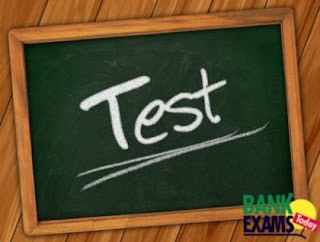# Mixed Aptitude Quiz - 3 (For SBI Clerical 2016)#### Ques 1.

In an examination paper of five question, 5% of the candidates answered all of them and 5% answered none. Of the rest, 25% candidates answered only one question and 20% answered 4 questions. If 396 candidates answered either 2 questions or 3 questions, the number of candidates that appeared for the examination was:
Solution :

Either 2 or 3 questions answered = 100- (5+5+22.5+18)
= 49.5%

⇒ 49.5% ---- 396
⇒ 100%  ---- 800

Number of persons appeared for exam = 800

#### Ques 2.

If a man walks 3 km/h, he is late to his office by 20 min. If he increases his speed to 6 km/h, he reaches the office 30 min early. The distance of his office from the starting place is
Solution  :

⇒ (X)/3  -  (X)/6  = (20+30)/60
⇒ X/6 = 5/6
⇒ Distance (X) = 5 Km

Note : In both cases time is  late or early   should be taken difference between two cases .

#### Ques 3.

Two trains started at the time one from A to B and other from B to A. If they arrived at B and A, respectively 4 h and 9 h after they passed each other, the ratio of the speeds of the two trains was
Solution :
⇒ Time = 4:9
⇒ Speed = √9:√4  = 3:2

#### Ques 4.

2cm of rain has fallen on a square km of land.assuming that 50% of the rain drops could have been collected and contained in a pool having a 100m*10m base,by what level has the water in the pool increased ?
Solution :

⇒ Pool area=100m×10m=1000
⇒ Volume rain= (1km)²×2cm=1000×1000×2/100=20000

⇒ 100%-----------20000
⇒ 50%   -----------10000
⇒ Level increased = volume/area
10000/1000 = 10m increased

#### Ques 5.

An amount of Rs. 2430 is divided among A, B and C such that if their shares be reduced by Rs. 5, Rs.10 and Rs. 15 respectively, the remainders shall be in the ratio of 3 : 4 : 5. Then, B’s share was.?
Solution :
⇒ 5-3=2
⇒ 10-4=6
⇒ 15-5=10
⇒ 2+6+10=18 parts

⇒ 18 parts ------ 2430
⇒ 6 parts.  ------- 810

B share is 810 rupees

#### Ques 6.

The average marks in science subject of a class of 20 students is 68 .if the marks of two students were misread as 48 and 65 instead ogre the actual marks 72 and 61 respectively what would be the correct average
Solution :

20 people average marks =68

⇒ 48+65=113
⇒ 72+61=133

⇒ Increased marks =133-113 = 20
⇒ Due to this 20 marks overall average increased = 20/20 =1
Correct average = 68+1=69

#### Ques 7.

Speed of boat A is 2km/hr less than speed of boat B. Time taken by boat A to travel a distance of 20 km downstream is 30 min more than time taken by B to travel the same distance downstream. If the speed of the current is 1/3rd of the speed of boat A, what is the speed of boat B?
Solution :
⇒ Speed of boat "A"   =x
⇒ Speed of boat  "B"  =x+2
⇒ Speed of Stream      =x/3
⇒ 20/(x+x/3) - 30/(x+2+x/3)=1/2
X = 6
Speed of boat "B" = 8 kmph

#### Ques 8.

The front wheels of a wagon are 2π feet in circumference and the rear wheels are 3π feet in circumference. When the front wheels have made 10 more revolutions than the rear wheels, how many feet has the wagon travelled?
Solution :
⇒ 2π(x+10)= 3πx
X=20
3π*20=60π

#### Ques 9.

A jar has 40 litres milk. From the jar, 8 litres of milk was taken out and replaced with an equal quantity of water. If 8 litres of the newly formed mixture is taken out of the jar, what is the final quantity of milk left in the jar?
Solution :
Tricky formula ---   final quantity =  n ( 1 - x/n)^t

Here    n --- Initial quantity
x --- quantity taken out
t ---  Number of times taken out milk and replaced water

Final quantity of milk = 40(1-8/40)^2 = 25.6lit

#### Ques 10.

Two mobile phones were purchased at the same price. One was sold at a profit of 20% and another was sold at a price which was Rs.1520 less than the price at which the first was sold. If the overall profit earned by selling both the mobile phones was 1% , what was the cost price of one mobile phone?
Solution :

Assume CP of two mobile = 100x+100x=200x

Total SP two mobiles =  200×101%=202x

One sold at 120x

Another at 202x-120x=82x

⇒ 120x - 82x = 1520

38x=1520
X =40

CP of mobile = 100x = 100*40 = 4000